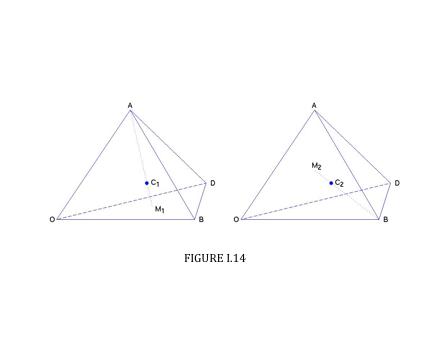$$\require{cancel}$$

# 1.7: Uniform Solid Tetrahedron, Pyramid and Cone

•• Contributed by Jeremy Tatum
• Emeritus Professor (Physics & Astronomy) at University of Victoria

Definition

A median of a tetrahedron is a line from a vertex to the centroid of the opposite face.

Theorem I.

The four medians of a tetrahedron are concurrent at a point 3/4 of the way from a vertex to the centroid of the opposite face.

Theorem II

The centre of mass of a uniform solid tetrahedron is at the meet of the medians.

Theorem I can be derived by a similar vector geometric argument used for the plane triangle. It is slightly more challenging than for the plane triangle, and it is left as an exercise for the reader. I draw two diagrams (Figure I.14). One shows the point $$\text{C}_{1}$$ that is 3/4 of the way from the vertex $$\text{A}$$ to the centroid of the opposite face. The other shows the point $$\text{C}_{2}$$ that is 3/4 of the way from the vertex $$\text{B}$$ to the centroid of its opposite face. You should be able to show that

$${\bf \text{C}_{1}}=({\bf\text{A+B+D}})/{4}$$In fact this suffices to prove Theorem I, because, from the symmetry between $${\bf \text{A}}$$, $${\bf \text{B}}$$ and $${\bf \text{D}}$$, one is bound to arrive at the same expression for the three-quarter way mark on any of the four medians. But for reassurance you should try to show, from the second figure, that

$${\bf \text{C}_{2}={\bf\text{A+B+D}})/{4}$$

The argument for Theorem II is easy, and is similar to the corresponding argument for plane triangles.

## Pyramid.

A right pyramid whose base is a regular polygon (for example, a square) can be considered to be made up of several tetrahedra stuck together. Therefore the centre of mass is 3/4 of the way from the vertex to the mid point of the base.

## Cone.

A right circular cone is just a special case of a regular pyramid in which the base is a polygon with an infinite number of infinitesimal sides. Therefore the centre of mass of a uniform right circular cone is 3/4 of the way from the vertex to the centre of the base.

We can also find the position of the centre of mass of a solid right circular cone by calculus. We can find its volume by calculus, too, but we'll suppose that we already know, from the theorem of Pappus, that the volume is $$\frac{1}{3}$$ × base × height.Consider the cone in Figure I.15, generated by rotating the line $$y= \frac{ax}{h}$$ (between $$x = 0$$ and $$x = h$$) through 360o about the $$x$$ axis. The radius of the elemental slice of thickness $$dx$$ at $$x$$ is $$\frac{ax}{h}$$. Its volume is $$\frac{\pi a^{2}x^{2} \delta x }{h^2}$$.

Since the volume of the entire cone is $$\frac{\pi a^{2}h}{3}$$, the mass of the slice is

$$M \times \frac{ \pi a^{2}x^{2} \delta x}{h^{2}} \div \frac{\pi a^{2}h}{3} = \frac{3Mx^{2}\delta x}{h^3}$$

where $$M$$ is the total mass of the cone. The first moment of mass of the elemental slice with respect to the $$y$$ axis is $$\frac{3Mx^{3}dx}{h^{3}}$$ .

The position of the centre of mass is therefore

$$\overline{x} = \frac{3}{h^{3}} \int_0^h x^3 dx = \frac{3}{4} h$$